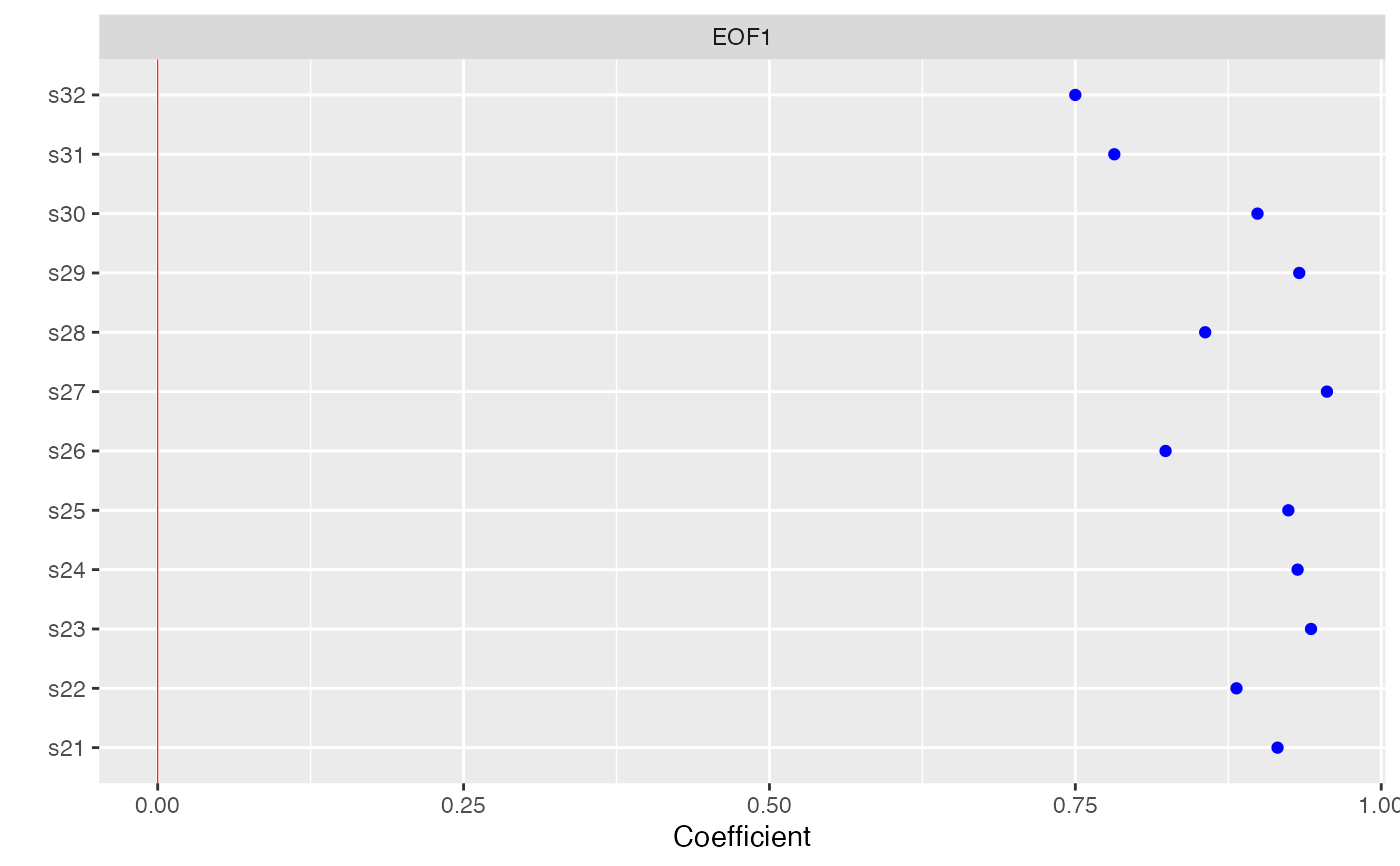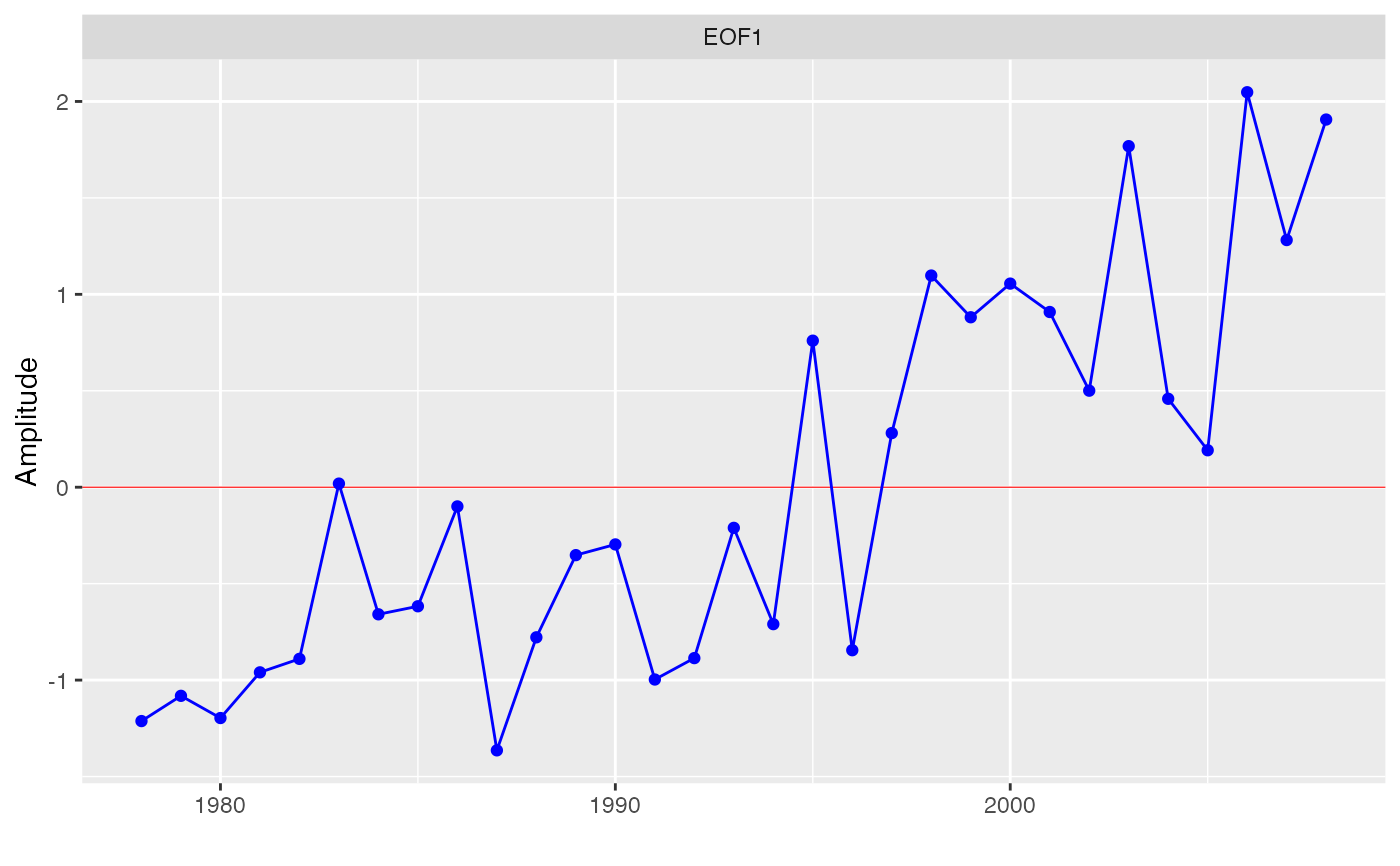Plots the rotated empirical orthogonal functions or amplitude time series resulting from eof.

eofPlot(x, type = c("coef", "amp"), rev = FALSE, ord = FALSE)

## Arguments

x result of the function eof whether the EOF coefficients or amplitudes should be plotted logical indicating whether coefficients and amplitudes should be multiplied by -1 logical indicating whether coefficients should be ordered by size

## Value

A plot of the EOF coefficients or amplitudes.

## Details

When the columns of the original data have a natural order, such as stations along a transect or months of the year, there may be no need to reorder the EOF coefficients. But if there is no natural order, such as when columns represents disparate sites around the world, the plot can be more informative if coefficients are ordered by size (ord = TRUE).

Coefficients and amplitudes for a given EOF may be more easily interpreted if rev = TRUE, because the sign of the first coefficient is arbitrarily determined and all the other signs follow from that choice.

eof

## Author

Alan Jassby, James Cloern

## Examples


# Create an annual matrix time series
chla1 <- aggregate(sfbayChla, 1, mean, na.rm = TRUE)
chla1 <- chla1[, 1:12]  # remove stations with missing years

# eofNum (see examples) suggests n = 1
e1 <- eof(chla1, n = 1)
eofPlot(e1, type = 'coef')eofPlot(e1, type = 'amp')